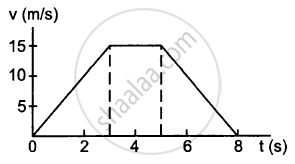Advertisement Remove all ads

# A Particle of Mass 50 G Moves in a Straight Line. the Variation of Speed with Time is Shown in Figure (5−E1). Find the Force Acting on the Particle at T = 2, 4 and 6 Seconds. - Physics

Sum

A particle of mass 50 g moves in a straight line. The variation of speed with time is shown in the following figure. Find the force acting on the particle at t = 2, 4 and 6 seconds.Advertisement Remove all ads

#### Solution

Given:
Mass of the particle, m = 50 g = 5 × 10−2 kg
Slope of the v-t graph gives acceleration.
At t = 2 s,
Slope = $\frac{15}{3} = 5 m/ s^2$
So, acceleration, a = 5 m/s2
F = ma = 5 × 10−2 × 5
⇒ F = 0.25 N along the motion.

At t = 4 s,
Slope = 0
So, acceleration, a = 0
⇒ F = 0

At t = 6 sec,
Slope =$\frac{- 15}{3} = - 5 m/ s^2$
So, acceleration, a = − 5 m/s2
F = ma = − 5 × 10−2 × 5
⇒ F = − 0.25 N along the motion
or, F = 0.25 N opposite the motion.

Is there an error in this question or solution?
Advertisement Remove all ads

#### APPEARS IN

HC Verma Class 11, 12 Concepts of Physics 1
Chapter 5 Newton's Laws of Motion
Q 6 | Page 79
Advertisement Remove all ads
Advertisement Remove all ads
Share
Notifications

View all notifications

Forgot password?